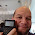Saturday, October 9, 2021

Swiss Micros DM41X and HP Prime: Rounding Numbers to One Significant Digit

Swiss Micros DM41X and HP Prime:  Rounding Numbers to One Significant Digit

Introduction

This blog entry deals with rounding numbers to one significant digit, that is the first non-zero numbers.  The general 5/4 rounding rules are used:  round up if you are next digit is 5 or more.

Examples:

72.28 rounds to 70

78.28 rounds to 80

0.0283 rounds to 0.03

Rob Eastaway, author of Maths On the Back of an Envelope (see source below), introduces the concept of Zequals.  Zequals is the practice of rounding numbers to one significant digit, with it's symbol similar to the symbol for the constellation Aquarius the Water Bearer ( ♒ ).  The aim for rounding numbers to one significant digit is to aid in estimating arithmetic operations and make mental mathematics easier.

Is it always spot on?

The program RONE (Round to ONE Significant Digit) rounds positive, non-zero numbers to one significant digit.   An algorithm to do so may look like this:

Number:  x

a = log(x)

If a≥0, then a=int(a)

If a<0, then a=int(a)-1

n = int(x / (10^a) + 1/2) * 10^a

We can also use:

n = round(x / (10^a),0) * 10^a

I have included the HP Prime PPL code for RONE.  RONEARITH for the HP Prime compares the actual versus rounded calculation for the four arithmetic functions.

Swiss Micros DM41X Program:  RONE

01 LBL^T ZONE

02 CF 01

03 ENTER

04 LOG

05 X<0?

06 SF 01

07 INT

08 FS? 01

09 -

10 FS? 01

11 -

12 10^X

13 /

14 LASTX

15 X<>Y

16 2

17 1/X

18 +

19 INT

20 *

21 CF 01

22 RTN

23 END

Examples:

52.4564 XEQ RONE returns 50.0000

0.06834 XEQ RONE returns 0.0700

HP Prime Program:  RONE

EXPORT RONE(n)

BEGIN

// Round to one significant digit

LOCAL a;

a:=LOG(n);

IF a<0 THEN

a:=IP(a)-1;

ELSE

a:=IP(a);

END;

RETURN ROUND(n/ALOG(a),0)*ALOG(a);

END;

HP Prime Program:  RONEARITH

EXPORT RONEARITH()

BEGIN

// 2021-07-26 EWS

// Input form

LOCAL c,x,y,r,t,l;

l:={"+","-",CHAR(#D7h),CHAR(#F7h)};

INPUT({x,y,{c,l}},

"1 Sig Digit Arithmetic",

{"x: ","y: ","OP: "},

{"x>0","y>0","Arithmetic Operation"});

PRINT();

IF c==1 THEN

PRINT(STRING(x)+"+"+STRING(y));

r:=ZR(x)+ZR(y);

t:=x+y;

END;

IF c==2 THEN

PRINT(STRING(x)+"-"+STRING(y));

r:=ZR(x)-ZR(y);

t:=x-y;

END;

IF c==3 THEN

PRINT(STRING(x)+CHAR(#D7h)+STRING(y));

r:=ZR(x)*ZR(y);

t:=x*y;

END;

IF c==4 THEN

PRINT(STRING(x)+CHAR(#F7h)+STRING(y));

r:=ZR(x)/ZR(y);

t:=x/y;

END;

PRINT("Estimate: "+STRING(r));

PRINT("Actual: "+STRING(t));

END;

ZR(n)

BEGIN

// Round to one significant digit

LOCAL a;

a:=LOG(n);

IF a<0 THEN

a:=IP(a)-1;

ELSE

a:=IP(a);

END;

RETURN ROUND(n/ALOG(a),0)*ALOG(a);

END;

Source:

Eastaway, Rob.   Maths On the Back of an Envelope  HarperCollins Publishers:  Dublin, Ireland  3rd Ed.  2021.  ISBN 978-0-00-844449-5

Eddie

All original content copyright, © 2011-2021.  Edward Shore.   Unauthorized use and/or unauthorized distribution for commercial purposes without express and written permission from the author is strictly prohibited.  This blog entry may be distributed for noncommercial purposes, provided that full credit is given to the author.

1.Hello again Eddie, I like to read your blog and help me start a new week.

It looks like we share a lot of common interests in simple maths and numbers crashing with actual or vintage pockets tools ! ;)

I also like to explore and share discoveries; by rounding numbers to one significant digit on my old (but trusty) HP-41C, I found that we don't have to use any program at all; just turn display format to ENG 0 !

I this makes my day, I like this good blog...

1.Thank you for the tip, C.Ret.

Numworks Update 17.2 - Highlights

Numworks Update 17.2 - Highlights Update is available at: https://www.numworks.com/ The Numworks website will detect your calculator and wi...